# Chapter 7 Coordinate Geometry NCERT Exemplar Solutions Exercise 7.2 Class 10 Maths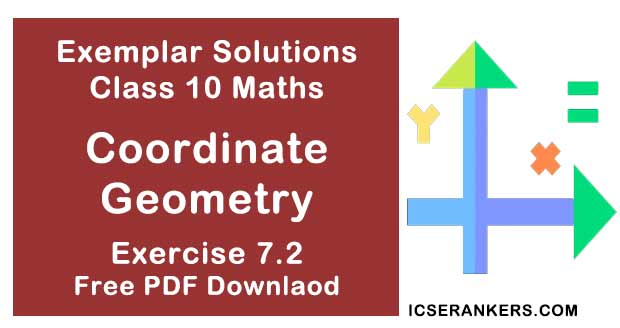Chapter Name NCERT Maths Exemplar Solutions for Chapter 7 Coordinate Geometry Exercise 7.2 Book Name NCERT Exemplar for Class 10 Maths Other Exercises Exercise 7.1Exercise 7.3Exercise 7.4 Related Study NCERT Solutions for Class 10 Maths

### Exercise 7.2 Solutions

State whether the following statements are true or false. Justify your answer.

1. ΔABC with vertices A (-2,0), B(2, 0) and C(0, 2) is similar to ΔDEF with vertices D(-4, 0), E(4, 0) and F(0, 4).
Solution
True.
Explanation :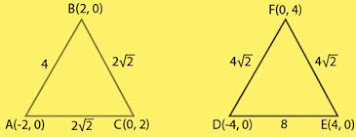By distance formula ,
d = √[(x2 – x1)2 + (y2 – y1)2 ]
we can find,Also,FD = 4√2
So,
AB/DE = BC/EF = CA/FD = 1/2
Hence, triangle ABC and DEF are similar.

2. Point P(-4, 2) lies on the line segment joining the points A(-4, 6) and B(-4, -6).
Solution
True,
Explanation :
We will plot the points P(-4, 2), A(-4, 6) and B(-4, -6)on a graph paper and connect the points.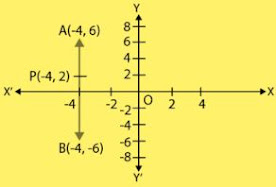So, from the graph it is clear that, point P(-4, 2) lies on the line segment joining the points A(-4, 6) and B(-4, -6)

3. The points (0, 5), (0, –9) and (3, 6) are collinear.
Solution
False
Explanation,
The points are collinear if area of a triangle formed by its points is equals to the zero.
Given,
x1 = 0,
x2 = 0,
x3 =  3 and
y1 = 5,
y2 = -9,
y3 = 6
Area of trianlge,
= ½ [ x1(y2 – y3 ) + x2 (y3 – y1) + x3 (y1 – y2) ]
= 1/2 [0(-9 - 6) - 0(6 - 5) + 4(5 + 9)]
= (1/2) (42)
= 42/2 ≠ 0
So, the points are not collinear.
From the above equation, it is clear that the points are not collinear.

4. Point P (0, 2) is the point of intersection of y–axis and perpendicular bisector of line segment joining the points A (–1, 1) and B (3, 3).
Solution
False
Explanation :
The points lying on perpendicular bisector of the line segment joining the two points is equidistant from the two points.
So,
PA should be equals to the PB.
Using distance formula,
d = √[(x2 – x1)2 + (y2 – y1)2 ]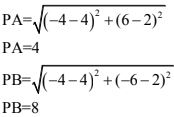So, PA is not equal to PB.

5. Points A (3, 1), B (12, –2) and C (0, 2) cannot be the vertices of a triangle.
Solution
True,
Explanation :
Coordinates of A = (x1 , y1 )
= (3, 1)
Coordinates of B = (x2 , y2 )
= (12, -2)
Coordinates of C = (x3 , y3 )
= (0, 2)
Area of ΔABC = ½ [ x1(y2 – y3 ) + x2 (y3 – y1) + x3 (y1 – y2) ]
= 1/2 [3 (-2-2) + 12(2 - 1) + 0{1 - (-2)}]
= 1/2 [3(-4) + 12(1) + 0(3)]
= 1/2 [-12 + 12 + 0]
= 1/2 × 0 = 0
Area of ΔABC = 0
As, the points A(3, 1), B(12, -2) and C(0, 2) are collinear.
So, the points A(3, 1), B(12, -2) and C(0, 2) can't be the vertices of a triangle.

6. Points A (4, 3), B (6, 4), C (5, –6) and D (–3, 5) are the vertices of a parallelogram.
Solution
False
Explanation :
The given points are A(4, 3), B(6, 4), C(5, -6) and D(-3, 5).
We will find the distances AB, BC, CD and AD respectively by distance formula.
We will see that,
Every distance is different from one another.
As the distances are different, we can conclude that the points are not the vertices of a parallelogram.

7. A circle has its center at the origin and a point P (5, 0) lies on it. The point Q (6, 8) lies outside the circle.
Solution
True
At first, we draw a circle and a point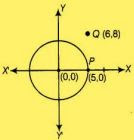Also, if the distance of any point from the centre is less than/equal to/ more than the radius, then the point is inside/on/outside the circle, respectively
OP = √[(5-0)2 + (0 - 0)2 ]
⇒ OP = √25
⇒ OP = 5 units
which is radius of the circle
and,
OQ = √[(6-0)2 + (8 - 0)2 ]
⇒ OQ = √(100)
⇒ OQ = 10 units
We see that, OQ > OP
So, it is true that point Q(6, 8), lies outside the circle.

8. The point A (2, 7) lies on the perpendicular bisector of line segment joining the points P (6, 5) and Q (0, – 4).
Solution
False
If A(2, 7) lies on perpendicular bisector of P(6, 5) and Q(0, -4) then AP = AQ
AP = √[(6-2)2 + (5 - 7)2 ]
⇒ AP = √(16 + 4)
⇒ AP = √20 units
and,
AQ = √[(0-2)2 + (-4 - 7)2 ]
⇒ AQ = √125 units
Therefore, A does not lies on the perpendicular bisector of PQ.

9. Point P(5, -3) is one of the two points of trisection of the line segment joining the point A(7, -2) and B(1, -5).
Solution
Let the point P(5, -3) divides the line segment joining the points A(7, -2) and B(1, -5) in the ratio k : 1 internally.
By section formula, the coordinate of point P will be,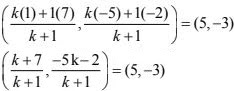So,
(k + 7)/(k + 1) = 5
⇒ k + 7 = 5(k + 1)
⇒ -4k = -2
⇒ k = 1/2
The point P divides the line segment AB in ratio 1 : 2.
Therefore, point P in the point of trisection of AB.

10. Points A(-6, 10), B(-4, 6) and C(3, -8) are collinear such that AB = (2/9)AC.
Solution
True
If the area of triangle formed by the points (x1 , y1 ),  (x2 , y2 ) and  (x3 , y3 ) is zero, then the points are collinear,
ar(ABC) = ½ [ x1(y2 – y3 ) + x2 (y3 – y1) + x3 (y1 – y2) ] = 0
= 1/2 [-6{6 - (-8)} -4(-8 - 10) + 3(10 - 6)] = 0
= -84 + 84 = 0
So, it is true,
And the points are collinear.
Also,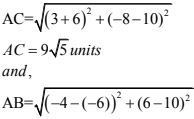AB = 2√5 units
Now,
AB/AC = 2√5/9√5
⇒ AB = (2/9) AC
Which is the required relation .

11. The point P (-2, 4) lies on a circle of radius 6 and centre C(3, 5).
Solution
False
If the distance between the centre and any point is equal to the radius, then we say that point lie on the circle.
So, distance between P(-2, 4) and centre (3, 5)
Distance  = √[(3 + 2)2 + (5 - 4)2]
= √26
It is not equal to the radius of the circle.
So, the point P(-2, 4) does not lies on the circle.

12. The points A(-1, -2), B(4, 3) , C(2, 5) and D(-3, 0) in that order form a rectangle.
Solution
True,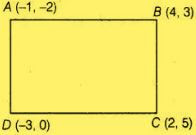Distance between A(-1, -2) and B(4, 3) is :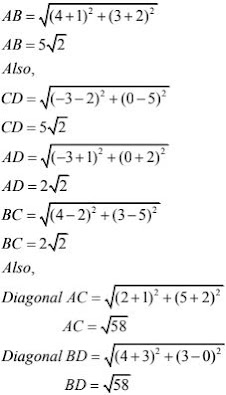As, opposite sides and diagonals AC and BD are equal.
Hence, the points A(-1 , -2), B(4, 3), C(2, 5) and D(-3, 0) form a rectangle.